# Algebra 1 : Factoring Polynomials

## Example Questions

1 2 3 4 5 6 7 8 9 11 Next →

### Example Question #411 : Polynomials

Factor the following polynomial: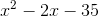.

Possible Answers: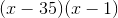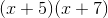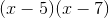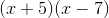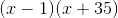Correct answer:Explanation:

Because theterm doesn’t have a coefficient, you want to begin by looking at theterm (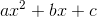) of the polynomial: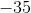.  Find the factors ofthat when added together equal the second coefficient (theterm) of the polynomial.

There are only four factors of: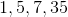, and only two of those factors,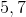, can be manipulated to equalwhen added together and manipulated to equalwhen multiplied together: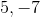(i.e.,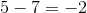).

### Example Question #101 : Factoring Polynomials

Factor the following polynomial: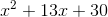.

Possible Answers: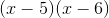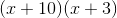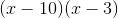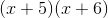Correct answer:Explanation:

Because theterm doesn’t have a coefficient, you want to begin by looking at theterm () of the polynomial:Find the factors ofthat when added together equal the second coefficient (theterm) of the polynomial:There are seven factors of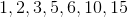, and only two of those factors,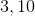, can be manipulated to equalwhen added together and manipulated to equalwhen multiplied together: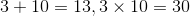### Example Question #11 : How To Factor A Variable

Solve forwhen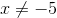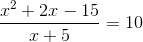Possible Answers: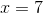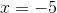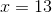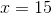Correct answer:Explanation:

First, factor the numerator: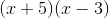.

Now your expression looks like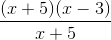Second, cancel the "like" terms -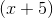- which leaves us with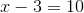.

Third, solve for, which leaves you with### Example Question #11 : How To Factor A Variable

Factor the following polynomial: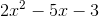.

Possible Answers: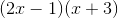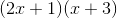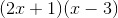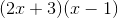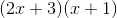Correct answer:Explanation:

Because theterm has a coefficient, you begin by multiplying theand theterms () together: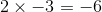Find the factors ofthat when added together equal the second coefficient (theterm) of the polynomial:There are four factors of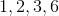, and only two of those factors,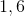, can be manipulated to equalwhen added together and manipulated to equalwhen multiplied together: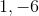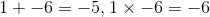### Example Question #422 : Polynomials

Factor: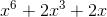Possible Answers: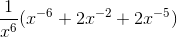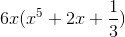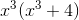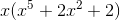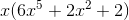Correct answer:Explanation:

For each term in this expression, we will notice that each shares a variable of.  This can be pulled out as a common factor.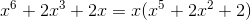There are no more common factors, and this is the reduced form.

The answer is:### Example Question #11 : How To Factor A Variable

Factor: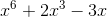Possible Answers: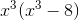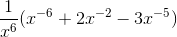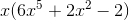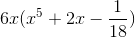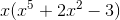Correct answer:Explanation:

For each term in this expression, we will notice that each shares a variable of.  This can be pulled out as a common factor.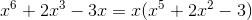There are no more common factors, and this is the reduced form.

The answer is:1 2 3 4 5 6 7 8 9 11 Next →

### All Algebra 1 Resources